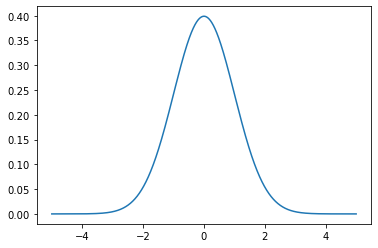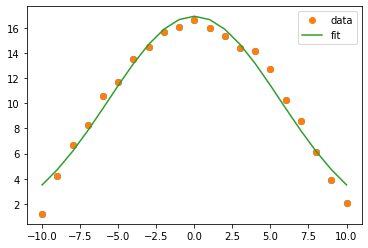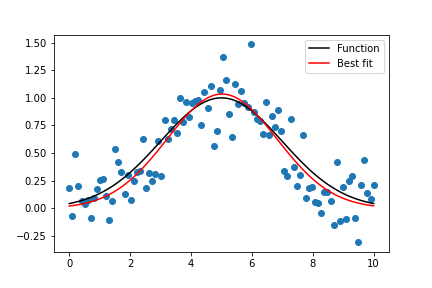# Python – Gaussian fit

• Difficulty Level : Medium
• Last Updated : 19 Nov, 2021

## What is normal or Guassian distribution?

When we plot a dataset such as a histogram, the shape of that charted plot is what we call its distribution. The most commonly observed shape of continuous values is the bell curve, also called the Gaussian or normal distribution.

It is named after the German mathematician Carl Friedrich Gauss. Some common example datasets that follow Gaussian distribution are Body temperature, People’s height, Car mileage, IQ scores.

Attention geek! Strengthen your foundations with the Python Programming Foundation Course and learn the basics.

To begin with, your interview preparations Enhance your Data Structures concepts with the Python DS Course. And to begin with your Machine Learning Journey, join the Machine Learning - Basic Level Course

Let’s try to generate the ideal normal distribution and plot it using Python.

### How to plot Gaussian distribution in Python

We have libraries like Numpy, scipy, and matplotlib to help us plot an ideal normal curve.

## Python3

 `import` `numpy as np``import` `scipy as sp``from` `scipy ``import` `stats``import` `matplotlib.pyplot as plt` `## generate the data and plot it for an ideal normal curve` `## x-axis for the plot``x_data ``=` `np.arange(``-``5``, ``5``, ``0.001``)` `## y-axis as the gaussian``y_data ``=` `stats.norm.pdf(x_data, ``0``, ``1``)` `## plot data``plt.plot(x_data, y_data)`

Output:The points on the x-axis are the observations, and the y-axis is the likelihood of each observation.

We generated regularly spaced observations in the range (-5, 5) using np.arange(). Then we ran it through the norm.pdf() function with a mean of 0.0 and a standard deviation of 1, which returned the likelihood of that observation. Observations around 0 are the most common, and the ones around -5.0 and 5.0 are rare. The technical term for the pdf() function is the probability density function.

### The Gaussian function:

First, let’s fit the data to the Gaussian function. Our goal is to find the values of A and B that best fit our data. First, we need to write a python function for the Gaussian function equation. The function should accept the independent variable (the x-values) and all the parameters that will make it.

## Python3

 `#Define the Gaussian function``def` `gauss(x, H, A, x0, sigma):``    ``return` `H ``+` `A ``*` `np.exp(``-``(x ``-` `x0) ``*``*` `2` `/` `(``2` `*` `sigma ``*``*` `2``))`

We will use the function curve_fit from the python module scipy.optimize to fit our data. It uses non-linear least squares to fit data to a functional form. You can learn more about curve_fit by using the help function within the Jupyter notebook or scipy online documentation.

The curve_fit function has three required inputs: the function you want to fit, the x-data, and the y-data you fit. There are two outputs. The first is an array of the optimal values of the parameters. The second is a matrix of the estimated covariance of the parameters from which you can calculate the standard error for the parameters.

Example 1:

## Python3

 `from` `__future__ ``import` `print_function``import` `numpy as np``import` `matplotlib.pyplot as plt``from` `scipy.optimize ``import` `curve_fit``xdata ``=` `[ ``-``10.0``, ``-``9.0``, ``-``8.0``, ``-``7.0``, ``-``6.0``, ``-``5.0``, ``-``4.0``, ``-``3.0``, ``-``2.0``, ``-``1.0``, ``0.0``, ``1.0``, ``2.0``, ``3.0``, ``4.0``, ``5.0``, ``6.0``, ``7.0``, ``8.0``, ``9.0``, ``10.0``]``ydata ``=` `[``1.2``, ``4.2``, ``6.7``, ``8.3``, ``10.6``, ``11.7``, ``13.5``, ``14.5``, ``15.7``, ``16.1``, ``16.6``, ``16.0``, ``15.4``, ``14.4``, ``14.2``, ``12.7``, ``10.3``, ``8.6``, ``6.1``, ``3.9``, ``2.1``]` `# Recast xdata and ydata into numpy arrays so we can use their handy features``xdata ``=` `np.asarray(xdata)``ydata ``=` `np.asarray(ydata)``plt.plot(xdata, ydata, ``'o'``)` `# Define the Gaussian function``def` `Gauss(x, A, B):``    ``y ``=` `A``*``np.exp(``-``1``*``B``*``x``*``*``2``)``    ``return` `y``parameters, covariance ``=` `curve_fit(Gauss, xdata, ydata)` `fit_A ``=` `parameters[``0``]``fit_B ``=` `parameters[``1``]` `fit_y ``=` `Gauss(xdata, fit_A, fit_B)``plt.plot(xdata, ydata, ``'o'``, label``=``'data'``)``plt.plot(xdata, fit_y, ``'-'``, label``=``'fit'``)``plt.legend()`Example 2:

## Python3

 `import` `numpy as np``from` `scipy.optimize ``import` `curve_fit``import` `matplotlib.pyplot as mpl` `# Let's create a function to model and create data``def` `func(x, a, x0, sigma):``    ``return` `a``*``np.exp(``-``(x``-``x0)``*``*``2``/``(``2``*``sigma``*``*``2``))` `# Generating clean data``x ``=` `np.linspace(``0``, ``10``, ``100``)``y ``=` `func(x, ``1``, ``5``, ``2``)` `# Adding noise to the data``yn ``=` `y ``+` `0.2` `*` `np.random.normal(size``=``len``(x))` `# Plot out the current state of the data and model``fig ``=` `mpl.figure()``ax ``=` `fig.add_subplot(``111``)``ax.plot(x, y, c``=``'k'``, label``=``'Function'``)``ax.scatter(x, yn)` `# Executing curve_fit on noisy data``popt, pcov ``=` `curve_fit(func, x, yn)` `#popt returns the best fit values for parameters of the given model (func)``print` `(popt)` `ym ``=` `func(x, popt[``0``], popt[``1``], popt[``2``])``ax.plot(x, ym, c``=``'r'``, label``=``'Best fit'``)``ax.legend()``fig.savefig(``'model_fit.png'``)`

Output:My Personal Notes arrow_drop_up